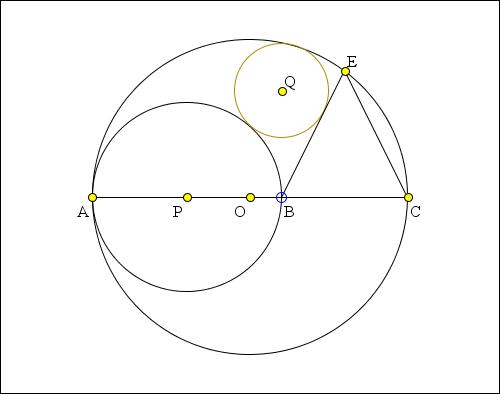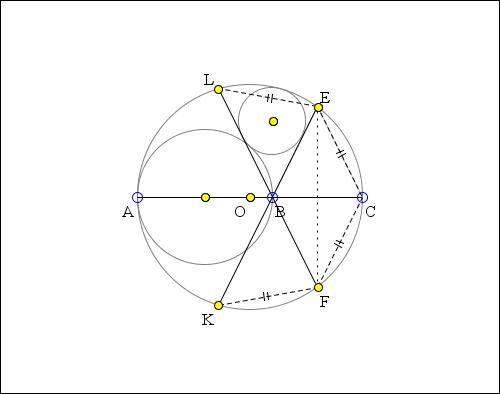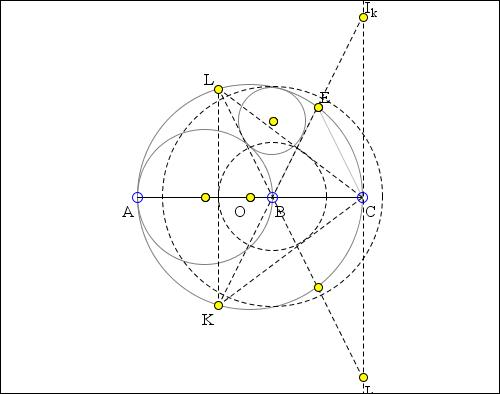Tangent Circles and an Isosceles Triangle II Solution by Vladimir

The applet presents a solution to the following Sangaku problem: Given a circle S with center O and diameter AC and point B on AC. Form circle G with center P and diameter AB and an isosceles triangle BCE with E on the circle S. Circle W with center Q is inscribed in the curvilinear triangle formed by circles S and G and the line BE. Prove that QB is perpendicular to AC.

(Another solution appears elsewhere. The one below is remarkable in an absolute absence of calculations.)

What if applet does not run? -->Reformulate the problem a little: (O), (P) are circles with diameters AC > AB tangent at A. Perpendicular bisector of BC cuts (O) at E, F, so that triangle BEC is isosceles (or BECF is a rhombus). Circle (Q) is internally / externally tangent to (O), (P) and to BE. Prove BQ is perpendicular to AC.EB, FB cut (O) again at K, L. FE bisects angle ∠CFL = ∠CFB, implying EL = EC = EB so that B is the incenter of ΔCKL. Perpendicular to AC at B cuts (O) at M, N. By construction, (O) cuts circle (B) with center B and diameter MN in diametrically opposite points which means that the inversion in (B) with negative power -BM² takes (O) into itself.Line EBK through the inversion center also goes to itself. Power of B to (O) is -BM² = BM × BN = BA × BC, meaning that the inversion takes A to C. Circle (P) with diameter AB goes to line perpendicular to AC at C, the external bisector of the angle ∠KCL.Let Ik, Il be K-, L-excenters of ΔCKL. The inverse image (Q') of (Q) is tangent to IkIl, to BE = BIk, and (by Feuerbach's Theorem) externally tangent to the 9-point circle (O) of the triangle BIkIl, therefore (Q') is its excircle in the angle BIkIl, centered on the external bisector of the angle EBF, i.e., the angle IkBIl, perpendicular to the internal bisector BC of this angle, therefore BQQ' is perpendicular to AC.This permits generalization to a non-isosceles triangle CKL with circumcircle (O) and incenter B and angle bisector CBA. (P) is circle with diameter AB, (Q) is circle internally tangent to (O), externally tangent to (P), and to the angle bisector KBE. No change in the proof that (O) is also tangent to the other angle bisector LBF.

Another solution makes use of the common inversion.Inversion - Introduction